# Kaggle Competition | Titanic: Machine Learning From a Disaster¶### Competition Description¶

The sinking of the RMS Titanic is one of the most infamous shipwrecks in history. On April 15, 1912, during her maiden voyage, the Titanic sank after colliding with an iceberg, killing 1502 out of 2224 passengers and crew. This sensational tragedy shocked the international community and led to better safety regulations for ships.

One of the reasons that the shipwreck led to such loss of life was that there were not enough lifeboats for the passengers and crew. Although there was some element of luck involved in surviving the sinking, some groups of people were more likely to survive than others, such as women, children, and the upper-class.

In this challenge, we ask you to complete the analysis of what sorts of people were likely to survive. In particular, we ask you to apply the tools of machine learning to predict which passengers survived the tragedy.

Goal

It is your job to predict if a passenger survived the sinking of the Titanic or not. For each PassengerId in the test set, you must predict a 0 or 1 value for the Survived variable.

Metric

Your score is the percentage of passengers you correctly predict. This is known simply as "accuracy”.

### Steps taken in this Notebook¶

1. Data Wrangling
• Visualizing missing data with MissingNo
• Checking correlation between survival and individual factors

2. Feature Engineering
• Feature engineering of new columns
• Passenger Title
• Family Size
• Travelling Alone?

3. Imputing Missing Data Values
• Randomizing the two missing Embarked values
• Imputing the missing fare value using the average fare paid by each Pclass
• Testing various ML Regression algorithms to predict age
• Linear Regression
• Bayesian Ridge
• Multilayer Perceptron (MLP)
• Decision Tree
• Bagging
• Using Multilayer Perceptron (MLP) to predict the missing age values

4. Predict Survival

• Test various ML Classifier algorithms to predict Survival

• Logistic Regression
• Support Vector
• Decision Tree
• Random Forest
• Multilayer Perceptron (MLP)
• K-Nearest Neighbours (KNN)
• Using MLP to predict Survival

• Formatting output for submission

### Required Libraries¶

• Numpy
• Pandas
• Missingno
• Seaborn
• Matplotlib
• Random
• SciKit-Learn
In [ ]:



## Data Wrangling¶

In :
import numpy as np
import pandas as pd
import random
import seaborn as sns
import matplotlib.pyplot as plt
from scipy.stats import ks_2samp, randint
%matplotlib inline

from sklearn.model_selection import train_test_split, RandomizedSearchCV
from sklearn.preprocessing import StandardScaler

from sklearn.neural_network import MLPRegressor, MLPClassifier
from sklearn.linear_model import LinearRegression, BayesianRidge, LogisticRegression
from sklearn.tree import DecisionTreeRegressor, DecisionTreeClassifier
from sklearn.ensemble import BaggingRegressor, RandomForestClassifier, AdaBoostClassifier
from sklearn.metrics import mean_squared_error, mean_absolute_error, accuracy_score
from sklearn.neighbors import KNeighborsClassifier
from sklearn.svm import SVC

all_dat = [train_dat, test_dat]

##### Training Dataset¶
In :
display(train_dat.head(3))
display(train_dat.info())
display(train_dat.describe())

PassengerId Survived Pclass Name Sex Age SibSp Parch Ticket Fare Cabin Embarked
0 1 0 3 Braund, Mr. Owen Harris male 22.0 1 0 A/5 21171 7.2500 NaN S
1 2 1 1 Cumings, Mrs. John Bradley (Florence Briggs Th... female 38.0 1 0 PC 17599 71.2833 C85 C
2 3 1 3 Heikkinen, Miss. Laina female 26.0 0 0 STON/O2. 3101282 7.9250 NaN S
<class 'pandas.core.frame.DataFrame'>
RangeIndex: 891 entries, 0 to 890
Data columns (total 12 columns):
PassengerId    891 non-null int64
Survived       891 non-null int64
Pclass         891 non-null int64
Name           891 non-null object
Sex            891 non-null object
Age            714 non-null float64
SibSp          891 non-null int64
Parch          891 non-null int64
Ticket         891 non-null object
Fare           891 non-null float64
Cabin          204 non-null object
Embarked       889 non-null object
dtypes: float64(2), int64(5), object(5)
memory usage: 83.6+ KB

None
PassengerId Survived Pclass Age SibSp Parch Fare
count 891.000000 891.000000 891.000000 714.000000 891.000000 891.000000 891.000000
mean 446.000000 0.383838 2.308642 29.699118 0.523008 0.381594 32.204208
std 257.353842 0.486592 0.836071 14.526497 1.102743 0.806057 49.693429
min 1.000000 0.000000 1.000000 0.420000 0.000000 0.000000 0.000000
25% 223.500000 0.000000 2.000000 20.125000 0.000000 0.000000 7.910400
50% 446.000000 0.000000 3.000000 28.000000 0.000000 0.000000 14.454200
75% 668.500000 1.000000 3.000000 38.000000 1.000000 0.000000 31.000000
max 891.000000 1.000000 3.000000 80.000000 8.000000 6.000000 512.329200
##### Testing Dataset¶
In :
display(test_dat.head(3))
display(test_dat.info())
display(test_dat.describe())

PassengerId Pclass Name Sex Age SibSp Parch Ticket Fare Cabin Embarked
0 892 3 Kelly, Mr. James male 34.5 0 0 330911 7.8292 NaN Q
1 893 3 Wilkes, Mrs. James (Ellen Needs) female 47.0 1 0 363272 7.0000 NaN S
2 894 2 Myles, Mr. Thomas Francis male 62.0 0 0 240276 9.6875 NaN Q
<class 'pandas.core.frame.DataFrame'>
RangeIndex: 418 entries, 0 to 417
Data columns (total 11 columns):
PassengerId    418 non-null int64
Pclass         418 non-null int64
Name           418 non-null object
Sex            418 non-null object
Age            332 non-null float64
SibSp          418 non-null int64
Parch          418 non-null int64
Ticket         418 non-null object
Fare           417 non-null float64
Cabin          91 non-null object
Embarked       418 non-null object
dtypes: float64(2), int64(4), object(5)
memory usage: 36.0+ KB

None
PassengerId Pclass Age SibSp Parch Fare
count 418.000000 418.000000 332.000000 418.000000 418.000000 417.000000
mean 1100.500000 2.265550 30.272590 0.447368 0.392344 35.627188
std 120.810458 0.841838 14.181209 0.896760 0.981429 55.907576
min 892.000000 1.000000 0.170000 0.000000 0.000000 0.000000
25% 996.250000 1.000000 21.000000 0.000000 0.000000 7.895800
50% 1100.500000 3.000000 27.000000 0.000000 0.000000 14.454200
75% 1204.750000 3.000000 39.000000 1.000000 0.000000 31.500000
max 1309.000000 3.000000 76.000000 8.000000 9.000000 512.329200

#### Let's examine the data briefly:¶

The Name, Sex, Ticket, Cabin, and Embarked are listed as strings The remaining lists (PassengerId, Age, SibSp, Parch, and Fare) are reported as integers, but some of them represent categories of values. These nominal values must be converted to dummy variables when creating models

Many of the columns have some missing values, and are difficult to conceptualize when reported in a table form (using info()). We will use the missingno package to make it easier to visualize where the missing values lie, and what proportions are the data are missing

### Visualizing missing data with missingno¶

In :
import missingno as msno
display(msno.bar(train_dat))
display(msno.bar(test_dat))
train_dat.drop(['Cabin', 'Ticket'], axis=1, inplace=True)
test_dat.drop(['Cabin', 'Ticket'], axis=1, inplace=True)

<matplotlib.axes._subplots.AxesSubplot at 0x10dafd31b70>
<matplotlib.axes._subplots.AxesSubplot at 0x10dabdd2c88>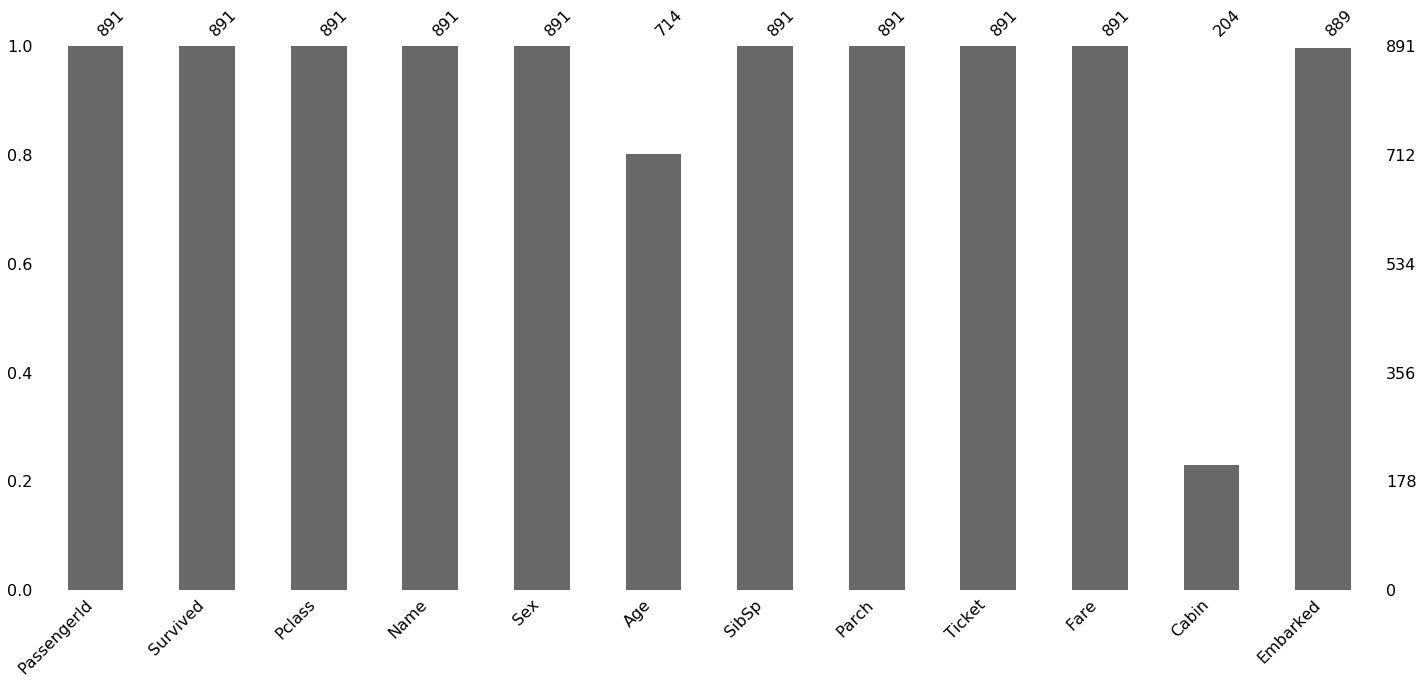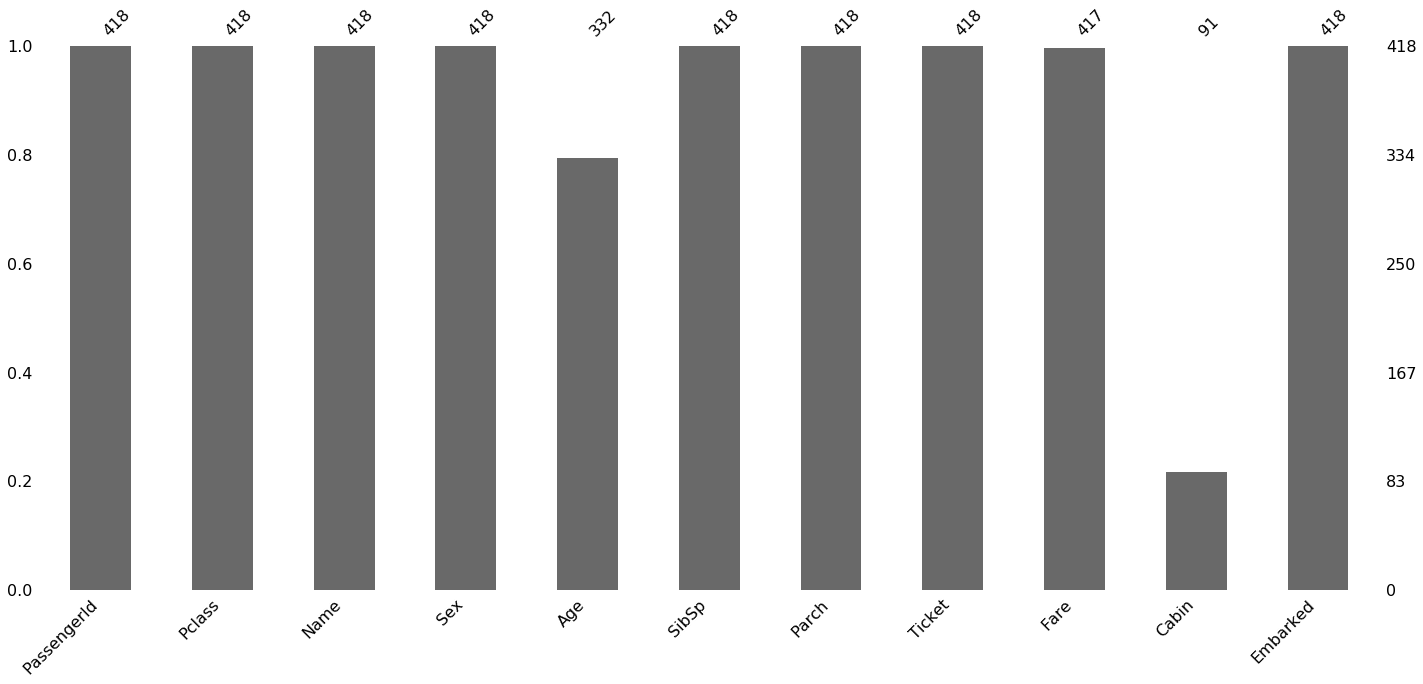In the Cabin column, we only have approximately 20% of values present in both datasets. There are too many missing values to attempt to impute the values. As such, we will drop it from the analysis

The Age column is missing approximately 20% of the values, but there are enough values present to impute the missing values. We will create an entire machine learning regression model for the missing age values later on

The training dataset contains two missing values for the Embarked data. Since there are only three possible options, we will randomly select values to fill the missing values

The test dataset contains one missing value for the Fare, which we will impute later based on the Pclass

### Checking correlation between survival and categorical factors¶

In :
train_dat.corr()

Out:
PassengerId Survived Pclass Age SibSp Parch Fare
PassengerId 1.000000 -0.005007 -0.035144 0.036847 -0.057527 -0.001652 0.012658
Survived -0.005007 1.000000 -0.338481 -0.077221 -0.035322 0.081629 0.257307
Pclass -0.035144 -0.338481 1.000000 -0.369226 0.083081 0.018443 -0.549500
Age 0.036847 -0.077221 -0.369226 1.000000 -0.308247 -0.189119 0.096067
SibSp -0.057527 -0.035322 0.083081 -0.308247 1.000000 0.414838 0.159651
Parch -0.001652 0.081629 0.018443 -0.189119 0.414838 1.000000 0.216225
Fare 0.012658 0.257307 -0.549500 0.096067 0.159651 0.216225 1.000000

#### Pclass¶

In :
train_dat[['Pclass', 'Survived']].groupby('Pclass').mean()

Out:
Survived
Pclass
1 0.629630
2 0.472826
3 0.242363

#### Sex¶

In :
train_dat[['Sex', 'Survived']].groupby('Sex').mean()

Out:
Survived
Sex
female 0.742038
male 0.188908

#### Age¶

In :
age_survived = train_dat.loc[train_dat['Survived'] == 1]['Age'].dropna()

fig = sns.kdeplot(age_survived, label='Survived', clip=[0,80])
fig.set(xlim=(0, 80))


Out:
Ks_2sampResult(statistic=0.0887768379960963, pvalue=0.12555581240058969)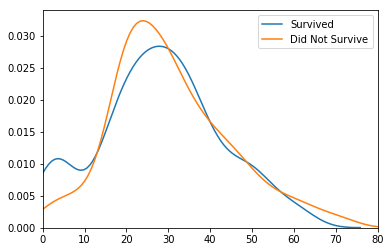#### Sibsp¶

In :
sns.barplot(train_dat['SibSp'], train_dat['Survived'])
display(train_dat[['Parch', 'Survived']].groupby('Parch').mean())

Survived
Parch
0 0.343658
1 0.550847
2 0.500000
3 0.600000
4 0.000000
5 0.200000
6 0.000000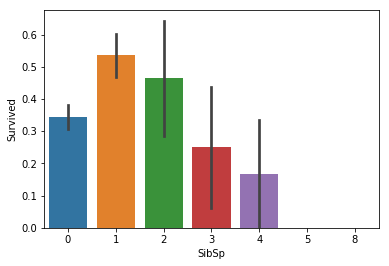#### Parch¶

In :
sns.barplot(train_dat['Parch'], train_dat['Survived'])
display(train_dat[['Parch', 'Survived']].groupby('Parch').mean())

Survived
Parch
0 0.343658
1 0.550847
2 0.500000
3 0.600000
4 0.000000
5 0.200000
6 0.000000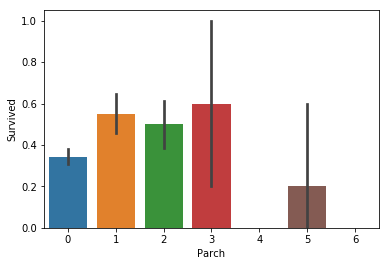#### Fare¶

In :
fare_survived = train_dat.loc[train_dat['Survived'] == 1]['Fare'].dropna()

fig = sns.kdeplot(fare_survived, label='Survived', clip=[0,80])
fig.set(xlim=(0, 80))

Out:
Ks_2sampResult(statistic=0.30236794171220405, pvalue=1.8678359776799793e-17)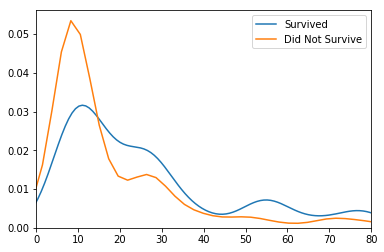In :
fig = plt.figure(figsize=(12,8))
sns.boxplot(train_dat['Pclass'], train_dat['Fare'])

Out:
<matplotlib.axes._subplots.AxesSubplot at 0x10dabfba860>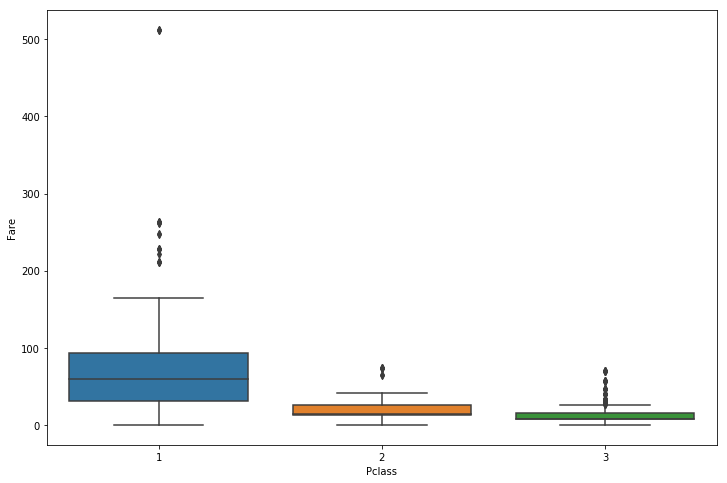## Feature Engineering¶

#### Extract Passenger Title¶

We will attempt to extract titles from the name

In :
import re as re
def title_extract_function(string):
title = re.search('([A-Za-z]+)\.', string)
return title

for df in all_dat:
df['Title'] = df['Name'].apply(title_extract_function)

display(pd.crosstab(all_dat['Title'], all_dat['Sex']))
display(pd.crosstab(all_dat['Title'], all_dat['Sex']))

Sex female male
Title
Capt. 0 1
Col. 0 2
Countess. 1 0
Don. 0 1
Dr. 1 6
Jonkheer. 0 1
Major. 0 2
Master. 0 40
Miss. 182 0
Mlle. 2 0
Mme. 1 0
Mr. 0 517
Mrs. 125 0
Ms. 1 0
Rev. 0 6
Sir. 0 1
Sex female male
Title
Col. 0 2
Dona. 1 0
Dr. 0 1
Master. 0 21
Miss. 78 0
Mr. 0 240
Mrs. 72 0
Ms. 1 0
Rev. 0 2

Some titles are held by a select few, and will be grouped into an "Other" category

In :
for df in all_dat:
df['Title'] = df['Title'].replace(['Mlle.', "Ms."], 'Miss.')
df['Title'] = df['Title'].replace(['Mme.'], 'Mrs.')
df['Title'] = df['Title'].replace(['Capt.','Col.','Countess.','Don.', 'Dona.','Dr.','Jonkheer.','Lady.', 'Major.', 'Rev.',
'Sir.'],'Other')

train_dat[['Title', 'Survived']].groupby('Title').mean()

Out:
Survived
Title
Master. 0.575000
Miss. 0.702703
Mr. 0.156673
Mrs. 0.793651
Other 0.347826

#### Family Size¶

In :
for df in all_dat:
df['Family Size'] = df['Parch'] + df['SibSp'] +1

train_dat[['Family Size', 'Survived']].groupby('Family Size').mean()

Out:
Survived
Family Size
1 0.303538
2 0.552795
3 0.578431
4 0.724138
5 0.200000
6 0.136364
7 0.333333
8 0.000000
11 0.000000

#### Travelling Alone?¶

In :
for df in all_dat:
df['Alone'] = 0
df.loc[df['Family Size'] == 1, 'Alone'] = 1
train_dat[['Alone', 'Survived']].groupby('Alone').mean()

Out:
Survived
Alone
0 0.505650
1 0.303538

### Imputing Missing Data Values¶

#### Embarked¶

In :
random.seed(1234)
while train_dat['Embarked'].isna().sum() > 0:
train_dat['Embarked'].fillna(random.randint(1,3), limit=1, inplace=True)
train_dat['Embarked'].replace({1:"S", 2:"C", 3:"Q"}, inplace=True)

train_dat[['Embarked', 'Survived']].groupby('Embarked').mean()

Out:
Survived
Embarked
C 0.556213
Q 0.389610
S 0.337984

#### Fare Class¶

In order to fill in the missing Fare value, we use the most correlated factor(s)

In :
Fare_corr = pd.DataFrame(train_dat.corr()['Fare'].drop(['Fare'],axis=0))
Fare_corr.reindex(Fare_corr.Fare.abs().sort_values(inplace=False, ascending=False).index)

Out:
Fare
Pclass -0.549500
Alone -0.271832
Survived 0.257307
Family Size 0.217138
Parch 0.216225
SibSp 0.159651
Age 0.096067
PassengerId 0.012658

The most significant factor that is correlated with Fare is the Pclass. We will impute the missing fare value based average value of the passenger's class

In :
sns.barplot(x=train_dat['Pclass'], y=train_dat['Fare'])

average_fare_by_class = test_dat.groupby(by=['Pclass'], as_index=False)['Fare'].mean()

if (test_dat.loc[test_dat['Fare'].isnull(),'Pclass'] == 3).values == True:
test_dat.loc[test_dat['Fare'].isnull(),'Fare'] = average_fare_by_class[average_fare_by_class['Pclass'] == 1]['Fare']
elif (test_dat.loc[test_dat['Fare'].isnull(),'Pclass'] == 2).values == True:
test_dat.loc[test_dat['Fare'].isnull(),'Fare'] = average_fare_by_class[average_fare_by_class['Pclass'] == 2]['Fare']
else:
test_dat.loc[test_dat['Fare'].isnull(),'Fare'] = average_fare_by_class[average_fare_by_class['Pclass'] == 3]['Fare']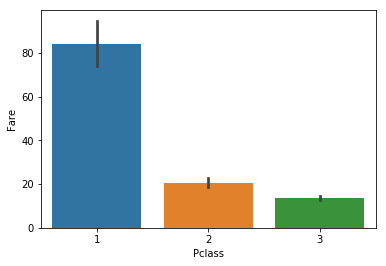Now that we have filled in the missing fare value, we can cut the range of Fares into distinct categorical groups. An easy way to visualize the distribution is through a violin plot.

In :
sns.violinplot(train_dat['Fare'])
max_fare = train_dat['Fare'].max()
plt.plot([60, 60], [-1, 1], linewidth=2)
plt.plot([110, 110], [-1, 1], linewidth=2)
plt.plot([180, 180], [-1, 1], linewidth=2)
plt.plot([max_fare, max_fare], [-1, 1], linewidth=2)

Out:
[<matplotlib.lines.Line2D at 0x10da5694b00>]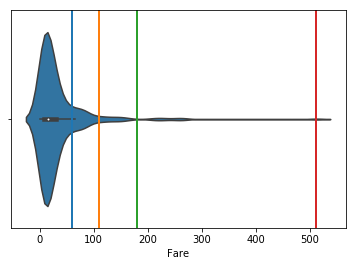We can use the plot to divide the Fares into four groups:

1. Fare Type 1 = \$0 - \$60
2. Fare Type 2 = \$61 - \$110
3. Fare Type 3 = \$111 - \$180
4. Fare Type 4 = \\$181+

Next, we need to test if the correlation between Fare Type and Survival

In :
for df in all_dat:
df['Fare Type'] = pd.cut(df['Fare'], [0, 60, 110,180, 1000], labels=['1', '2', '3', "4"])

train_dat[['Fare Type', 'Survived']].groupby('Fare Type').mean()

Out:
Survived
Fare Type
1 0.343501
2 0.616438
3 0.793103
4 0.700000

### Predicting the missing age values¶

In :
display(all_dat.head(2))

PassengerId Survived Pclass Name Sex Age SibSp Parch Fare Embarked Title Family Size Alone Fare Type
0 1 0 3 Braund, Mr. Owen Harris male 22.0 1 0 7.2500 S Mr. 2 0 1
1 2 1 1 Cumings, Mrs. John Bradley (Florence Briggs Th... female 38.0 1 0 71.2833 C Mrs. 2 0 2
PassengerId Pclass Name Sex Age SibSp Parch Fare Embarked Title Family Size Alone Fare Type
0 892 3 Kelly, Mr. James male 34.5 0 0 7.8292 Q Mr. 1 1 1
1 893 3 Wilkes, Mrs. James (Ellen Needs) female 47.0 1 0 7.0000 S Mrs. 2 0 1

#### Convert Categorical Data into Dummy Variables¶

In :
categorical_df = []
for df in all_dat:
sex = pd.get_dummies(df['Sex'],drop_first=True)
embark = pd.get_dummies(df['Embarked'],drop_first=True)
title = pd.get_dummies(df['Title'], drop_first=True)
fare = pd.get_dummies(df['Fare Type'], drop_first=True)
dummies = pd.concat([sex, embark, title, fare], axis=1)
df2 = df.drop(['Sex','Embarked','Name','Fare','Fare Type','Title'], axis=1, inplace=True)
df3 = pd.concat([df, sex, embark, title, fare], axis=1)
categorical_df.append(df3)

In [ ]:


In [ ]:



#### Separate the missing data from the complete data¶

In :
missing_age = categorical_df[pd.isnull(categorical_df).any(axis=1)].drop(['PassengerId'], axis=1)
complete_age = categorical_df.dropna().drop(['PassengerId'], axis=1)

In [ ]:



#### Find the optimal machine learning regression algorithm¶

Since we are predicting the numeric values of age, we calculated the accuracy of the model using the RMSE (root-mean-square error) and the MAE (mean absolute error).

The ML regression algorithms tested are:

1. Linear Regression
2. Bayesian Ridge
3. Multilayer Perceptron (MLP)
4. Decision Tree
5. Bagging Regressor



We repeated the testing of each algorithm 1,000 times with differen splits for the training and testing splits. We used the resulting averages of RMSE and MAE to determine the best algorithm to use to predict the missing age values. The resulting algorithm with the lowest RMSE/MAE values will be used

In :
total_RMSE = pd.DataFrame(columns=["LM RMSE", "BRR RMSE", "NNR RMSE", "DTR RMSE", "BR RMSE"])
total_MAE = pd.DataFrame(columns=["LM MAE", "BRR MAE", "NNR MAE", "DTR MAE", "BR MAE"])

for i in range(0, 100):
x = complete_age.drop('Age',axis=1)
y = complete_age['Age']
x_train, x_test, y_train, y_test = train_test_split(x, y, test_size=0.30)
scaler = StandardScaler()
scaler.fit(x_train)
x_train = scaler.transform(x_train)
x_test = scaler.transform(x_test)

lm = LinearRegression()
lm.fit(x_train, y_train)
lm_pred = lm.predict(x_test)

brr = BayesianRidge()
brr.fit(x_train, y_train)
brr_pred = brr.predict(x_test)

nnr = MLPRegressor(hidden_layer_sizes=(100,), activation='relu', solver='adam',    alpha=0.001,batch_size='auto',
learning_rate='constant', learning_rate_init=0.01, power_t=0.5, max_iter=1000, shuffle=True,
random_state=None, tol=0.0001, verbose=False, warm_start=False, momentum=0.9,
nesterovs_momentum=True, early_stopping=False, validation_fraction=0.1, beta_1=0.9, beta_2=0.999,
epsilon=1e-08)
nnr.fit(x_train, y_train)
nnr_pred = nnr.predict(x_test)

dtr = DecisionTreeRegressor()
dtr.fit(x_train,y_train)
dtr_pred = dtr.predict(x_test)

br = BaggingRegressor()
br.fit(x_train, y_train)
br_pred = br.predict(x_test)

RMSEs = [mean_squared_error(y_test, lm_pred), mean_squared_error(y_test, brr_pred), mean_squared_error(y_test, nnr_pred),
mean_squared_error(y_test, dtr_pred), mean_squared_error(y_test, br_pred)]
total_RMSE.loc[i] = RMSEs
MAEs = [mean_absolute_error(y_test, lm_pred), mean_absolute_error(y_test, brr_pred), mean_absolute_error(y_test, nnr_pred),
mean_absolute_error(y_test, dtr_pred), mean_absolute_error(y_test, br_pred)]
total_MAE.loc[i] = MAEs

average_RMSE = total_RMSE.mean(axis=0).values
average_MAE = total_MAE.mean(axis=0).values

Age_ML_Summary = pd.DataFrame(average_RMSE, columns=["RMSE"], index=['Linear Reg', "Bayesian Ridge", 'MLP',
'Decision Tree', 'Bagging Reg'])
Age_ML_Summary['MAE'] = average_MAE
Age_ML_Summary.transpose()

Out:
Linear Reg Bayesian Ridge MLP Decision Tree Bagging Reg
RMSE 1.190776e+24 126.890969 128.980617 163.670386 139.088605
MAE 1.289010e+10 8.889148 8.681706 9.596950 8.988091

The Multilayer Perceptron algorithm produced the lowest RMSE and MAE values, and will be used to predict the missing age values. But first, we need to tune the hyperparameters

### Using Multilayer Perceptron (MLP) to predict the missing age values¶

#### Predict missing age values using MLP¶

In :
def predict_age(df):
if (df['PassengerId'].max()== 891):
df = df.drop('Survived', axis=1)
missing_age = df[pd.isnull(df).any(axis=1)]
complete_age = df.dropna()

x_train = complete_age.drop(['Age', 'PassengerId'],axis=1)
y_train = complete_age['Age']
x_test = missing_age.drop(['Age', 'PassengerId'], axis=1)

scaler = StandardScaler()
scaler.fit(x_train)
x_train = scaler.transform(x_train)
x_test = scaler.transform(x_test)

nnr = MLPRegressor(hidden_layer_sizes=(100,), activation='relu', solver='adam', alpha=0.001,batch_size='auto',
learning_rate='constant', learning_rate_init=0.01, power_t=0.5, max_iter=1000, shuffle=True,
random_state=1234, tol=0.0001, verbose=False, warm_start=False, momentum=0.9,
nesterovs_momentum=True, early_stopping=False, validation_fraction=0.1, beta_1=0.9, beta_2=0.999,
epsilon=1e-08)
nnr.fit(x_train, y_train)
predicted_age = nnr.predict(x_test)

missing_age = df[pd.isnull(df).any(axis=1)].drop('Age',axis=1)
age_df = missing_age[['PassengerId']]
age_df = age_df.assign(Age = predicted_age)
age_df.sort_values(by=['PassengerId'])
return age_df

complete_data = []
for df in categorical_df:
pred_age = predict_age(df)
complete_df = df.combine_first(pred_age)
complete_data.append(complete_df)


### Predict Survival¶

#### Finding the optimal machine learning classifier algorithm¶

In :
x = complete_age.drop('Survived',axis=1)
y = complete_age['Survived']
x_train, x_test, y_train, y_test = train_test_split(x, y, test_size=0.30)
scaler = StandardScaler()
scaler.fit(x_train)
x_train = scaler.transform(x_train)
x_test = scaler.transform(x_test)


#### Randomized Search of Hyperparameters¶

In :
#import timeit
#start = timeit.default_timer()

total_accuracy = pd.DataFrame(columns=["Logistic Regression", "SVC", "Decision Tree",

for i in range(0, 5):
x = complete_age.drop('Survived',axis=1)
y = complete_age['Survived']
x_train, x_test, y_train, y_test = train_test_split(x, y, test_size=0.30)
scaler = StandardScaler()
scaler.fit(x_train)
x_train = scaler.transform(x_train)
x_test = scaler.transform(x_test)

params = {'C': np.arange(0.001, 100, 0.5)}
logreg_grid = RandomizedSearchCV(LogisticRegression(), params)
logreg_grid.fit(x_train, y_train)
logreg_pred = logreg_grid.predict(x_test)

params = {'C': np.arange(0.1, 5, 0.1), 'gamma': np.arange(0.00001, 1, 0.05), 'kernel': ['rbf', 'linear', 'sigmoid']}
svc_grid = RandomizedSearchCV(SVC(), params)
svc_grid.fit(x_train, y_train)
svc_predictions = svc_grid.predict(x_test)

params = {'criterion': ['gini', 'entropy'], 'max_depth': range(1,50)}
decisiontree_grid = RandomizedSearchCV(DecisionTreeClassifier(), params)
decisiontree_grid.fit(x_train, y_train)
DT_predictions = decisiontree_grid.predict(x_test)

params = {'criterion': ['gini', 'entropy'], 'n_estimators': range(5, 100), 'max_depth': range(1,50)}
rf_grid = RandomizedSearchCV(RandomForestClassifier(), params)
rf_grid.fit(x_train, y_train)
rf_predictions = rf_grid.predict(x_test)

params = {'learning_rate': np.arange(0.01, 1.1, 0.1), 'n_estimators': np.arange(50,200, 25)}

params = {'activation': ['identity', 'logistic', 'tanh', 'relu'], 'solver': ['lbfgs', 'sgd', 'adam'],
'alpha': [0.000001, 0.00001, 0.0001, 0.001, 0.01, 0.1]}
mlp_grid = RandomizedSearchCV(MLPClassifier(max_iter=1000),params)
mlp_grid.fit(x_train, y_train)
mlp_predictions = mlp_grid.predict(x_test)

params = {'n_neighbors': np.arange(5,50, 2), 'weights': ['uniform', 'distance']}
KN_grid = RandomizedSearchCV(KNeighborsClassifier(),params)
KN_grid.fit(x_train, y_train)
KN_predictions = KN_grid.predict(x_test)

accuracies = [accuracy_score(y_test, logreg_pred),accuracy_score(y_test, svc_predictions),
accuracy_score(y_test, DT_predictions), accuracy_score(y_test, rf_predictions),
accuracy_score(y_test, KN_predictions)]

total_accuracy.loc[i] = accuracies

average_accuracy = pd.DataFrame(total_accuracy.mean(axis=0), columns=['Accuracy'])
average_accuracy.transpose()

244.51487812747249

Out:
Logistic Regression SVC Decision Tree Random Forest Adaboost MLP Kneighbours
Accuracy 0.832558 0.824186 0.79907 0.817674 0.803721 0.826047 0.791628

Although the Logistic Regression algorithm resulted in the highest average accuracy over 100 runs, we will proceed with an MLP classifer anyways ¯\_(ツ)_/¯

#### Using an MLP Classifier for final survival predictions¶

In :
x_train = complete_data.drop(['Survived', 'PassengerId'], axis=1)
y_train = complete_data['Survived']
x_test = complete_data.drop(['PassengerId'], axis=1)

scaler = StandardScaler()
scaler.fit(x_train)
x_train = scaler.transform(x_train)
x_test = scaler.transform(x_test)

In :
mlp = MLPClassifier(max_iter=2000, random_state=1234)
mlp.fit(x_train, y_train)
final_mlp = mlp.predict(x_test)


#### Formatting output for submission¶

In :
final_submission = complete_data.copy()
final_submission['Survived'] = final_mlp
submission_df = pd.DataFrame()
submission_df[['PassengerId', 'Survived']] = final_submission[['PassengerId', 'Survived']]
display(submission_df.groupby('Survived').count())

submission_df.to_csv("Titanic Submission.csv", index=False)

PassengerId Survived
0 892 0
1 893 0
2 894 0
3 895 0
4 896 1
PassengerId
Survived
0 267
1 151